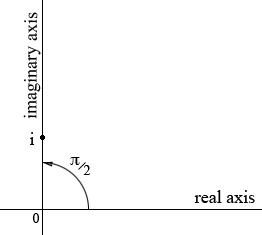SEARCH HOMEMath Central Quandaries & QueriesQuestion from randomness, a student: i have learnt that 'i' is square root of -1. What is then i^i ? It baffles my maths teacher... Thanks Randomness of piHi,

In many situations with complex numbers, especially when dealing with powers, it is helpful to express the numbers in exponential form. That is express the number as r eiθ = r(cos(θ) + i sin(θ)) where θ is expressed in radians. To do this I usually think of the geometric representation of the number in the complex plane. Using this technique the number i can be written

i = eiπ/2.Thus

ii = (eiπ/2)i = ei2π/2 = e-π/2

Thus ii is a real number! In decimal form it is approximately 0.207880.

Here is a more algebraic way to see it. De Moivre showed that eix = cosx + isinx so that eiπ/2 = cos(π/2) + isin(π/2) = i, for example. Thus to look at ii one could consider ii = (eiπ/2)i and that's the same as e(iπ/2)i = e(i2)(π/2) = e-π/2.

Penny

Hi,

Since sine and cosine are periodic with period 2π, for any integer n

ei(2nπ +π/2) = cos(π/2 + 2nπ) + isin(π/2 + 2nπ) = cos(π/2) + isin(π/2) = i

Hence ii has infinitely many values, e2nπ -π/2 for n any integer. The valuse closest unity being approximately

(..., 0.0003882, 0.2079, 111.32, 59609, ...)

RDMath Central is supported by the University of Regina and The Pacific Institute for the Mathematical Sciences.